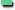Read a file word by word using DBMS_LOBOracle offers several possibilities to process file from within PL/SQL. The most used package is UTL_FILE, but with the disadvantage that the read-buffer is limited to 1023 bytes. If you want to read huge chunks of files you can use the DBMS_LOB package, even for the processing of plain ASCII files.

There are two solutions to read a file with DBMS_LOB

• The file is treaded as a large binary object LOB. The whole file is read and saved in a table column of the data type LOB and then processed.

• The file is read and processed directly from the filesystem location. This Tip shows exactly this case.

Example

Suppose we want to read a big file word by word directly from PL/SQL without saving the whole file in a table column. The words in the file are separated with a blank. For simplicity we assume, that there is exactly one blank between the words and the file is a stream with a newline at the end of the file.

First we have to create an ORACLE directory as the schema owner. Do not add a trailing "/" at the end of the directory path.

sqlplus scott/tiger
SQL> create directory READ_LOB_DIR as 'C:\Users\Zahn\Work';

Next we create the procedure READ_FILE_LOB, which reads the file word by word.

CREATE OR REPLACE
----------------------------------------------------------------
-- Read an ASCII file word by word with DBMS_LOB package.
--
-- Before you can use this procedure create an ORACLE directory
-- object as the owner of this peocedure.
--
-- sqlplus scott/tiger
-- SQL> create directory READ_LOB_DIR as 'C:\Users\Zahn\Work';
--
-- Do not add a trailing "/" at the end of the directory path.
--
----------------------------------------------------------------

-- Input Directory as specified in create directory

-- Input File which is read word by word
l_fil       CONSTANT VARCHAR2(30) := 'testfile.txt';

-- Separator Character between words is a BLANK (ascii = 32)
l_seb       CONSTANT RAW(100) := UTL_RAW.CAST_TO_RAW(CHR(32));

-- Character at the end of the file is NEWLINE (ascii = 10)
l_sen       CONSTANT RAW(100) := UTL_RAW.CAST_TO_RAW(CHR(10));

-- Pointer to the BFILE
l_loc       BFILE;

-- Current position in the file (file begins at position 1)
l_pos       NUMBER := 1;

-- Amount of characters have been read
l_sum       BINARY_INTEGER := 0;

l_buf       VARCHAR2(500);

-- End of the current word which will be read
l_end       NUMBER;

-- Return value
l_ret       BOOLEAN := FALSE;

BEGIN

-- Mapping the physical file with the pointer to the BFILE
l_loc := BFILENAME(l_dir,l_fil);

-- Check if the file exists
l_ret := DBMS_LOB.FILEEXISTS(l_loc) = 1;
IF (l_ret) THEN
dbms_output.put_line('File ' ||
l_fil || ' in Directory ' || l_dir || ' exists');

-- Open the file in READ_ONLY mode
LOOP

-- Calculate the end of the current word
l_end := DBMS_LOB.INSTR(l_loc,l_seb,l_pos,1);

-- Process end-of-file
IF (l_end = 0) THEN
l_end := DBMS_LOB.INSTR(l_loc,l_sen,l_pos,1);
l_sum := l_end - l_pos - 1;
dbms_output.put_line(UTL_RAW.CAST_TO_VARCHAR2(l_buf));
EXIT;
END IF;

l_sum := l_end - l_pos;
dbms_output.put_line(UTL_RAW.CAST_TO_VARCHAR2(l_buf));
l_pos := l_pos + l_sum + 1;
END LOOP;
DBMS_LOB.CLOSE(l_loc);
ELSE
dbms_output.put_line('File ' ||
l_fil || ' in Directory ' || l_dir || ' does not exist');
END IF;
EXCEPTION
WHEN OTHERS THEN
dbms_output.put_line('Error:' || SQLERRM);
DBMS_LOB.CLOSE(l_loc);
END;
/

The file testfile.txt has the following content

martin zahn seftigen

Output of the procedure

sqlplus scott/tiger